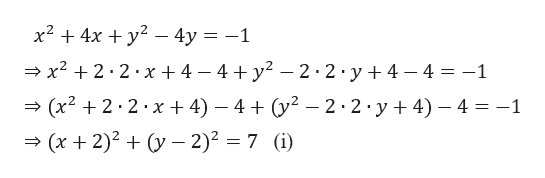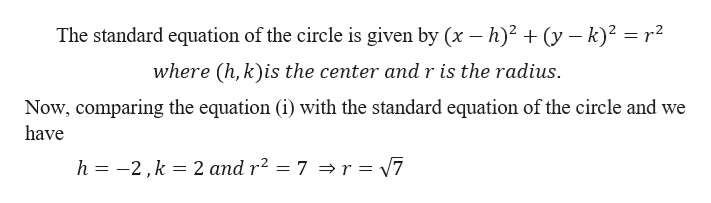# Draw a circle with an equation of x2+4x+y2−4y=1.

Question
1 views

Draw a circle with an equation of x2+4x+y2−4y=1.

check_circle

Step 1

Given,help_outlineImage Transcriptionclosex2 4x 4y = -1 x22.2 x + 4 - 4 + y2 - 2 2.y 4 4=-1 (x22 2 x 4) - 4 (y2-2 2 y4) 4 -1 . (x 2)y 2)2 7 (i fullscreen
Step 2

We know th...help_outlineImage TranscriptioncloseThe standard equation of the circle is given by (x - h)2 (y -k)2 r2 where (h, k)is the center andr is the radius Now, comparing the equation (i) with the standard equation of the circle and we have h -2,k 2 and r2 = 7 > r = 7 fullscreen

### Want to see the full answer?

See Solution

#### Want to see this answer and more?

Solutions are written by subject experts who are available 24/7. Questions are typically answered within 1 hour.*

See Solution
*Response times may vary by subject and question.
Tagged in

### Other